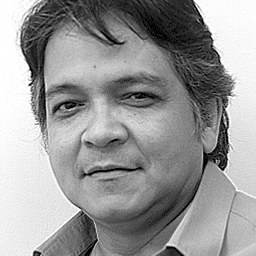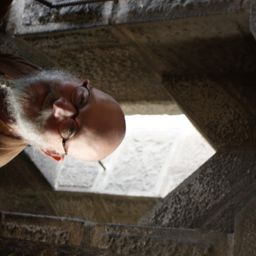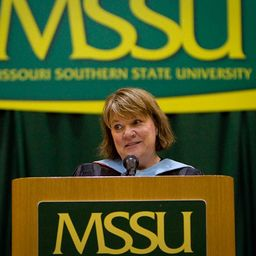💬 👋 We’re always here. Join our Discord to connect with other students 24/7, any time, night or day.Join Here!

## Educators+ 6 more educators

### Problem 1

Write an equation that expresses the fact that a function $f$ is continuous at the number 4.Ma. Theresa A.

### Problem 2

If $f$ is continuous on $(-\infty, \infty)$, what can you say about its graph?Leon D.

### Problem 3

(a) From the graph of $f$, state the numbers at which $f$ is discontinuous and explain why.
(b) For each of the numbers stated in part (a), determine whether $f$ is continuous from the right, or from the left, nor neither.

DM
David M.

### Problem 4

From the graph of $g$, state the intervals on which $g$ is continuous.

DM
David M.

### Problem 5

Sketch the graph of a function $f$ that is continuous except for the stated discontinuity.

Discontinuous, but continuous from the right, at 2.Leon D.

### Problem 6

Sketch the graph of a function $f$ that is continuous except for the stated discontinuity.

Discontinuities at -1 and 4, but continuous from the left at -1 and from the right of 4Leon D.

### Problem 7

Sketch the graph of a function $f$ that is continuous except for the stated discontinuity.

Removable discontinuity at 3, jump discontinuity at 5.Leon D.

### Problem 8

Sketch the graph of a function $f$ that is continuous except for the stated discontinuity.

Neither left nor right continuous at -2, continuous only from the left at 2.Babita K.

### Problem 9

The toll $T$ charged for driving on a certain stretch of a toll road is 5 dollars except during rush hours (between 7 AM and 10 AM and between 4 PM and 7 PM) when the toll is 7 dollars.
(a) Sketch a graph of $T$ as a function of the time $t$, measured in hours past midnight.
(b) Discuss the discontinuities of this function and their significance to someone who uses the road.

KS
Keyan S.

### Problem 10

Explain why each function is continuous or discontinuous.
(a) The temperature at a specific location as a function of time
(b) The temperature at a specific time as a function of the distance due west from New York City
(c) The altitude above sea level as a function of the distance due west from New York City
(d) The cost of a taxi ride as a function of the distance traveled
(e) The current in the circuit for the lights in a room as a function of timeMichael C.

### Problem 11

Use the definition of continuity and the properties of limits to show that the function is continuous at the given number $a$.

$f(x) = (x + 2x^3)^4, \hspace{5mm} a = -1$Stark L.

### Problem 12

Use the definition of continuity and the properties of limits to show that the function is continuous at the given number $a$.

$g(t) = \frac{t^2 + 5t}{2t + 1}, \hspace{5mm} a = 2$Ma. Theresa A.

### Problem 13

Use the definition of continuity and the properties of limits to show that the function is continuous at the given number $a$.

$p(v) = 2 \sqrt{3v^2 + 1}, \hspace{5mm} a = 1$Ma. Theresa A.

### Problem 14

Use the definition of continuity and the properties of limits to show that the function is continuous at the given number $a$.

$f(x) = 3x^4 - 5x + \sqrt{x^2 + 4}, \hspace{5mm} a = 2$Daniel J.

### Problem 15

Use the definition of continuity and the properties of limits to show that the function is continuous on the given interval.

$f(x) = x + \sqrt{x - 4}, \hspace{5mm} [4, \infty)$Leon D.

### Problem 16

Use the definition of continuity and the properties of limits to show that the function is continuous on the given interval.

$g(x) = \frac{x - 1}{3x + 6}, \hspace{5mm} (-\infty, -2)$Leon D.

### Problem 17

Explain why the function is discontinuous at the given number $a$. Sketch the graph of the function.

$f(x) = \frac{1}{x + 2} \hspace{55mm} a = -2$

AK
Anjali K.

### Problem 18

Explain why the function is discontinuous at the given number $a$. Sketch the graph of the function.

$f(x) = \left\{ \begin{array}{ll} \dfrac{1}{x + 2} & \mbox{if$ x \neq -2 $} \hspace{40mm} a = -2\\ 1 & \mbox{if$ x = -2 $} \end{array} \right.$Suman Saurav T.

### Problem 19

Explain why the function is discontinuous at the given number $a$. Sketch the graph of the function.

$f(x) = \left\{ \begin{array}{ll} x + 3 & \mbox{if$ x \le -1 $} \hspace{40mm} a = -1\\ 2^x & \mbox{if$ x > -1 $} \end{array} \right.$Leon D.

### Problem 20

Explain why the function is discontinuous at the given number $a$. Sketch the graph of the function.

$f(x) = \left\{ \begin{array}{ll} \dfrac{x^2 - x}{x^2 - 1} & \mbox{if$ x \neq 1 $} \hspace{40mm} a = 1\\ 1 & \mbox{if$ x = 1 $} \end{array} \right.$Leon D.

### Problem 21

Explain why the function is discontinuous at the given number $a$. Sketch the graph of the function.

$f(x) = \left\{ \begin{array}{ll} \cos x & \mbox{if$ x < 0 $}\\ 0 & \mbox{if$ x = 0 $} \hspace{42mm} a = 0\\ 1 - x^2 & \mbox{if$ x > 0 $} \end{array} \right.$

AK
Anjali K.

### Problem 22

Explain why the function is discontinuous at the given number $a$. Sketch the graph of the function.

$f(x) = \left\{ \begin{array}{ll} \dfrac{2x^2 - 5x - 3}{x - 3} & \mbox{if$ x \neq 3 $} \hspace{30mm} a = 3\\ 6 & \mbox{if$ x = 3 $} \end{array} \right.$Ma. Theresa A.

### Problem 23

How would you "remove the discontinuity" of $f$? In other words, how would you define $f(2)$ in order to make $f$ continuous at 2?

$f(x) = \dfrac {x^2 - x - 2}{x - 2}$Leon D.

### Problem 24

How would you "remove the discontinuity" of $f$? In other words, how would you define $f(2)$ in order to make $f$ continuous at 2?

$f(x) = \dfrac {x^3 - 8}{x^2 - 4}$Leon D.

### Problem 25

Explain, using Theorems 4, 5, 7, and 9, why the function is continuous at every number in its domain. State the domain.

$F(x) = \dfrac{2x^2 - x - 1}{x^2 + 1}$

DM
David M.

### Problem 26

Explain, using Theorems 4, 5, 7, and 9, why the function is continuous at every number in its domain. State the domain.

$G(x) = \dfrac{x^2 + 1}{2x^2 - x - 1}$Linda H.

### Problem 27

Explain, using Theorems 4, 5, 7, and 9, why the function is continuous at every number in its domain. State the domain.

$Q(x) = \dfrac{\sqrt{x - 2}}{x^3 - 2}$Linda H.

### Problem 28

Explain, using Theorems 4, 5, 7, and 9, why the function is continuous at every number in its domain. State the domain.

$R(t) = \dfrac{e^{\sin t}}{2 + \cos \pi t}$Daniel J.

### Problem 29

Explain, using Theorems 4, 5, 7, and 9, why the function is continuous at every number in its domain. State the domain.

$A(t) = \arcsin(1 + 2t)$

DM
David M.

### Problem 30

Explain, using Theorems 4, 5, 7, and 9, why the function is continuous at every number in its domain. State the domain.

$B(x) = \dfrac{\tan x}{\sqrt{4 - x^2}}$

DM
David M.

### Problem 31

Explain, using Theorems 4, 5, 7, and 9, why the function is continuous at every number in its domain. State the domain.

$\displaystyle M(x) = \sqrt{1 + \frac{1}{x}}$Linda H.

### Problem 32

Explain, using Theorems 4, 5, 7, and 9, why the function is continuous at every number in its domain. State the domain.

$N(r) = \tan^{-1}(1 + e^{-r^2})$Daniel J.

### Problem 33

Locate the discontinuities of the function and illustrate by graphing.

$y = \dfrac{1}{1 + e^{1/x}}$Daniel J.

### Problem 34

Locate the discontinuities of the function and illustrate by graphing.

$y = \ln (\tan^2 x)$Leon D.

### Problem 35

Use continuity to evaluate the limit.

$\displaystyle \lim_{x \to 2} x \sqrt{20 - x^2}$Daniel J.

### Problem 36

Use continuity to evaluate the limit.

$\displaystyle \lim_{x \to \pi} \sin(x + \sin x)$Leon D.

### Problem 37

Use continuity to evaluate the limit.

$\displaystyle \lim_{x \to 1} \ln\biggl( \dfrac{5 - x^2}{1 + x} \biggr)$Leon D.

### Problem 38

Use continuity to evaluate the limit.

$\displaystyle \lim_{x \to 4} 3^{\sqrt{x^2 - 2x - 4}}$Daniel J.

### Problem 39

Show that $f$ is continuous on $(-\infty, \infty )$.

$f(x) = \left\{ \begin{array}{ll} 1 - x^2 & \mbox{if$ x \le 1 $}\\ \ln x & \mbox{if$ x > 1 $} \end{array} \right.$Ma. Theresa A.

### Problem 40

Show that $f$ is continuous on $(-\infty, \infty )$.

$f(x) = \left\{ \begin{array}{ll} \sin x & \mbox{if$ x < \pi/4 $}\\ \cos x & \mbox{if$ x \ge \pi/4 $} \end{array} \right.$Daniel J.

### Problem 41

Find the numbers at which $f$ is discontinuous. At which of these numbers is $f$ continuous from the right, from the left, or neither? Sketch the graph of $f$.

$f(x) = \left\{ \begin{array}{ll} x^2 & \mbox{if$ x < -1 $}\\ x & \mbox{if$ -1 \le x < 1 $} \\ 1/x & \mbox{if$ x \ge 1 $} \end{array} \right.$Cindy R.

### Problem 42

Find the numbers at which $f$ is discontinuous. At which of these numbers is $f$ continuous from the right, from the left, or neither? Sketch the graph of $f$.

$f(x) = \left\{ \begin{array}{ll} 2^x & \mbox{if$ x \le 1 $}\\ 3 - x & \mbox{if$ 1 < x \le 4 $} \\ \sqrt{x} & \mbox{if$ x > 4 $} \end{array} \right.$Leon D.

### Problem 43

Find the numbers at which $f$ is discontinuous. At which of these numbers is $f$ continuous from the right, from the left, or neither? Sketch the graph of $f$.

$f(x) = \left\{ \begin{array}{ll} x + 2 & \mbox{if$ x < 0 $}\\ e^x & \mbox{if$ 0 \le x \le 1 $} \\ 2 - x & \mbox{if$ x > 1 $} \end{array} \right.$

AK
Anjali K.

### Problem 44

The gravitational force exerted by the planet Earth on a unit mass at a distance $r$ from the center of the planet is

$F(r) = \left\{ \begin{array}{ll} \frac{GMr}{R^3} & \mbox{if$ r < R $}\\ \frac{GM}{r^2} & \mbox{if$ r \ge R $} \end{array} \right.$

where $M$ is the mass of Earth, $R$ is its radius, and $G$ is the gravitational constant. Is $F$ a continuous function of $r$?Linda H.

### Problem 45

For what value of the constant $c$ is the function $f$ continuous on $(-\infty, \infty)$?

$f(x) = \left\{ \begin{array}{ll} cx^2 + 2x & \mbox{if$ x < 2 $}\\ x^3 - cx & \mbox{if$ x \ge 2 $} \end{array} \right.$Ma. Theresa A.

### Problem 46

Find the values of $a$ and $b$ that make $f$ continuous everywhere.

$f(x) = \left\{ \begin{array}{ll} \dfrac{x^2 - 4}{x - 2} & \mbox{if$ x < 2 $}\\ ax^2 - bx + 3 & \mbox{if$ 2 \le x < 3 $} \\ 2x - a + b & \mbox{if$ x \ge 3 $} \end{array} \right.$Ma. Theresa A.

### Problem 47

Suppose $f$ and $g$ are continuous functions such that $g(2) = 6$ and $\displaystyle \lim_{x \to 2} [3f(x) + f(x)g(x)] = 36$. Find $f(2)$.Ma. Theresa A.

### Problem 48

Let $f(x) = 1/x$ and $g(x) = 1/x^2$.

(a) Find $(f \circ g)(x)$.
(b) Is $f \circ g$ continuous everywhere? Explain.Daniel J.

### Problem 49

Which of the following functions $f$ has a removable discontinuity at $a$? If the discontinuity is removable, find a function $g$ that agrees with $f$ for $x \neq a$ and is continuous at $a$.

(a) $f(x) = \dfrac{x^4 -1}{x - 1}$, $a = 1$
(b) $f(x) = \dfrac{x^3 - x^2 - 2x}{x - 2}$, $a = 2$
(c) $f(x) = [ \sin x ]$, $a = \pi$Carolyn B.

### Problem 50

Suppose that a function $f$ is continuous on $[0, 1]$ except at 0.25 and that $f(0) = 1$ and $f(1) = 3$. Let $N = 2$. Sketch two possible graphs of $f$, one showing that $f$ might not satisfy the conclusion of the Intermediate Value Theorem and one showing that $f$ might still satisfy the conclusion of the Intermediate Value Theorem (even though it doesn't satisfy the hypothesis.)Daniel J.

### Problem 51

If $f(x) = x^2 + 10 \sin x$, show that there is a number $c$ such that $f(c) = 1000$.Leon D.

### Problem 52

Suppose $f$ is continuous on $[1, 5]$ and the only solutions of the equation $f(x) = 6$ are $x = 1$ and $x = 4$. If $f(2) = 8$, explain why $f(3) > 6$.Stark L.

### Problem 53

Use the Intermediate Value Theorem to show that there is a root of the given equation in the specified interval.

$x^4 + x - 3 = 0$, $(1, 2)$Stark L.

### Problem 54

Use the Intermediate Value Theorem to show that there is a root of the given equation in the specified interval.

$\ln x = x - \sqrt{x}$, $(2, 3)$Stark L.

### Problem 55

Use the Intermediate Value Theorem to show that there is a root of the given equation in the specified interval.

$e^x = 3 - 2x$, $(0, 1)$Leon D.

### Problem 56

Use the Intermediate Value Theorem to show that there is a root of the given equation in the specified interval.

$\sin x = x^2 - x$, $(1, 2)$Daniel J.

### Problem 57

(a) Prove that the equation has at least one real root.
(b) Use your calculator to find an interval of length 0.01 that contains a root.

$\cos x = x^3$Oswaldo J.

### Problem 58

(a) Prove that the equation has at least one real root.
(b) Use your calculator to find an interval of length 0.01 that contains a root.

$\ln x = 3 - 2x$Daniel J.

### Problem 59

(a) Prove that the equation has at least one real root.
(b) Use your graphing device to find the root correct to three decimal places.

$100e^{-x/100} = 0.01x^2$Leon D.

### Problem 60

(a) Prove that the equation has at least one real root.
(b) Use your graphing device to find the root correct to three decimal places.

$\arctan x = 1 - x$Daniel J.

### Problem 61

Prove, without graphing, that the graph of the function has at least two $x$-intercepts in the specified interval.

$y = \sin x^3$, $(1, 2)$

Anupa D.

### Problem 62

Prove, without graphing, that the graph of the function has at least two $x$-intercepts in the specified interval.

$y = x^2 - 3 + 1/x$, $(0, 2)$Daniel J.

### Problem 63

Prove that $f$ is continuous at $a$ if and only if
$$\lim_{h \to 0}f(a + h) = f(a)$$Daniel J.

### Problem 64

To prove that sine is continuous, we need to show that $\lim_{x \to a} \sin x = \sin a$ for every real number $a$. By Exercise 63 an equivalent statement is that $$\lim_{h \to 0} \sin (a + h) = \sin a$$.

Use (6) to show that this is true.Daniel J.

### Problem 65

Prove that cosine is a continuous function.Daniel J.

### Problem 66

(a) Prove Theorem 4, part 3.
(b) Prove Theorem 4, part 5.Daniel J.

### Problem 67

For what values of $x$ is $f$ continuous?
$$f(x) = \left\{ \begin{array}{ll} 0 & \mbox{if  x  is rational}\\ 1 & \mbox{if  x  is irrational} \end{array} \right.$$Daniel J.

### Problem 68

For what values of $x$ is $g$ continuous?
$$g(x) = \left\{ \begin{array}{ll} 0 & \mbox{if  x  is rational}\\ x & \mbox{if  x  is irrational} \end{array} \right.$$Daniel J.

### Problem 69

Is there a number that is exactly 1 more than its cube?Daniel J.

### Problem 70

If $a$ and $b$ are positive numbers, prove that the equation

$$\dfrac{a}{x^3 + 2x^2 - 1} + \dfrac{b}{x^3 + x - 2} = 0$$

has at least one solution in the interval $(-1, 1)$.Daniel J.

### Problem 71

Show that the function
$f(x) = \left\{ \begin{array}{ll} x^4 \sin (1/x) & \mbox{if$ x \neq 0 $}\\ 0 & \mbox{if$ x = 0 $} \end{array} \right.$

is continuous on $(-\infty, \infty)$.Daniel J.

### Problem 72

(a) Show that the absolute value function $F(x) = | x |$ is continuous everywhere.
(b) Prove that if $f$ is a continuous function on an interval, then so is $| f |$.
(c) Is the converse of the statement in part (b) also true? In other words, if $| f |$ is continuous, does it follow that $f$ is continuous? If so, prove it. If not, find a counterexample.Jimmy Y.